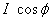## Monday, June 3, 2013

### Power and Power Factor

The component of current  which is in phase with the applied voltage () and contributes to active or true power of the circuit is known as active or watt-ful or in phase component of alternating current
and the component of current which is perpendicular to applied voltage () and contributes to reactive power of the circuit is known as reactive or watt-less component of alternating current.

The product of rms values of the current and voltage, VI is called the apparent power and is measured in volt-amperes or kilo- volt-amperes (kVA).

The true power in an ac circuit is obtained by multiplying the apparent power by the power factor and expressed in watts or kilo-watts (KW).It is also known as active power.

The product of apparent power and sine angle between voltage and current,is known as reactive power. This is also known as watt-less power and expressed in reactive volt-amperes or kilo volt-amperes reactive (kVAr).

And

The above relations can be easily be followed by referring to the power diagram as shown in fig.3.17 (b) above.

Power Factor:

Power factor may be defined as:
Cosine of the angle between voltage and current or
The ratio of the resistance and impedance or
The ratio of true power and apparent power.

The power factor can never be grater than unity. The power factor is expressed either as fraction or as percentage. It is useful practice to attach the word ‘leading’ or ‘lagging’ with the numerical value of power factor to signify whether the current leads or lag behind the voltage.

The power factor of an ac system should be as high as possible so that current and applied voltage are brought as near in phase as possible. Low power factor possesses the following drawbacks:
The current needed to obtain the given power is very high. This in turn results in the increased resistive losses thereby resulting in decreased efficiency.
It cause the poor voltage regulation
It limits the output of the generators and transformers due to high current.
Cause more power loss in transmission line.
It reduces the kWh, since the true (active) power is less.